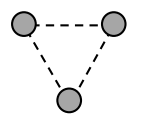# Problem: Three small 100-kg spheres are arranged as shown, forming a triangle of equal 10-cm sides. Calculate the magnitude and direction of the net force acting on the bottom sphere.

###### FREE Expert Solution
85% (299 ratings)
###### Problem Details

Three small 100-kg spheres are arranged as shown, forming a triangle of equal 10-cm sides. Calculate the magnitude and direction of the net force acting on the bottom sphere.Frequently Asked Questions

What scientific concept do you need to know in order to solve this problem?

Our tutors have indicated that to solve this problem you will need to apply the Gravitational Forces in 2D concept. You can view video lessons to learn Gravitational Forces in 2D. Or if you need more Gravitational Forces in 2D practice, you can also practice Gravitational Forces in 2D practice problems.